# ISEE Upper Level Quantitative : How to find the missing part of a list

## Example Questions

### Example Question #21 : Sets

The Fibonacci sequence begins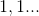with each subsequent term being the sum of the previous two.

Which is the greater quantity?

(a) The product of the seventh and eighth terms of the Fibonacci sequence

(b) The product of the sixth and ninth terms of the Fibonacci sequence

(a) and (b) are equal.

(b) is greater.

It is impossible to tell from the information given.

(a) is greater.

(a) is greater.

Explanation:

By starting with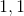and adding each pair of consecutive terms to get each subsequent term, we can generate the first nine terms of the Fibonacci sequence: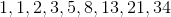(a) The seventh and eighth terms are 13 and 21; their product is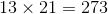.

(b) The sixth and ninth terms are 8 and 34; their product is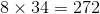.

(a) is greater.

### Example Question #22 : Sets

The first six terms of a sequence are as follows: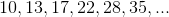Which is the greater quantity?

(a) The eighth term of the sequence

(b)(a) is greater.

It is impossible to tell from the information given.

(b) is greater.

(a) and (b) are equal.

(a) is greater.

Explanation:

Each successive term is derived by adding a quantity to the previous term that increments by 1 with each term: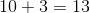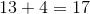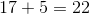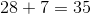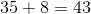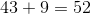, the eighth term, is greater than.

### Example Question #172 : Data Analysis

Seven freshmen and seven sophomores are running for student council. Each freshman will choose three of the candidates from his or her class; each sophomore will choose four from his or her class.

Which is the greater quantity?

(a) The number of ways a freshman can fill out his or her ballot

(b) The number of ways a sophomore can fill out his or her ballot

(b) is greater.

(a) is greater.

It is impossible to tell from the information given.

(a) and (b) are equal.

(a) and (b) are equal.

Explanation:

In both cases, candidates are selected without regard to order, making them combinations (as opposed to permutations).

(a) The freshmen are choosing three elements from a group of seven, or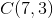: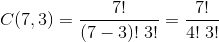(b) The sophomores are choosing four elements from a group of seven, or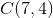: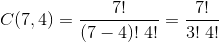These quantities are equal.

### Example Question #173 : Data Analysis

An arithmetic sequence begins as follows: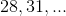The Fibonacci sequence begins,

with each subsequent term being the sum of the previous two.

Which is the greater quantity?

(a) The tenth term of the arithmetic sequence

(b) The tenth term of the Fibonacci sequence

(a) is greater.

(b) is greater.

(a) and (b) are equal.

It is impossible to tell from the information given.

(a) and (b) are equal.

Explanation:

(a) The arithmetic sequence has common difference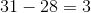; its tenth term is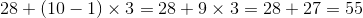.

(b) By beginning withand taking the sum of the previous two terms to get each successive term, we can generate the Fibonacci sequence: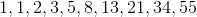Therefore the two are equal.

### Example Question #178 : Data Analysis

Twelve candidates are running for four vacancies on the school board. Six of the candidates are Democrats, and six are Republicans. Samantha wants to vote for two Democrats and two Republicans.

Which is the greater quantity?

(a) The number of ways Samantha can cast her ballot so that she votes for two Democrats and two Republicans

(b)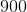It is impossible to tell from the information given.

(a) and (b) are equal.

(b) is greater.

(a) is greater.

(b) is greater.

Explanation:

The number of ways Samantha can choose two Democrats out of the six is the number of combinations of 2 chosen from a set of 6: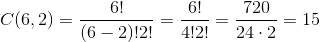This is also the number of ways Samantha can choose two Republicans out of the six.

These are two independent events, so we multiply them to get the number of total ways Samantha can vote with these specifications: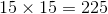Therefore (b) is greater.

### Example Question #179 : Data Analysis

The Fibonacci sequence begins,

with each subsequent term being the sum of the previous two.

Which is the greater quantity?

(a) The sum of the seventh, eighth, ninth, and tenth terms of the Fibonacci sequence

(b) The twelfth term of the Fibonacci sequence

(a) is greater.

It is impossible to tell from the information given.

(a) and (b) are equal.

(b) is greater.

(b) is greater.

Explanation:

By beginning withand taking the sum of the previous two terms to get each successive term, we can generate the Fibonacci sequence: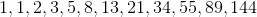The seventh through tenth terms, added together, yield a sum of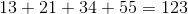,

which is less than the twelfth term,.

### Example Question #180 : Data Analysis

The Student Council comprises thirty students, from which four are chosen to fill the offices of President, Vice-President, Secretary, and Treasurer. Which is the greater quantity?

(a) The number of ways the four offices can be filled

(b)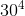It is impossible to tell from the information given.

(b) is greater.

(a) and (b) are equal.

(a) is greater.

(b) is greater.

Explanation:

(a) There are 30 ways to fill the office of President. Afterward, there will be 29 ways to fill the office of Vice-President, 28 ways to fill the office of Secretary, and 27 ways to fill the office of Treasurer. Multiply: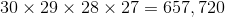(b)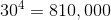This makes (b) greater.

### Example Question #21 : How To Find The Missing Part Of A List

The first six terms of a sequence are: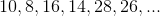Which is the greater quantity?

(a) The tenth term of the sequence

(b)(b) is greater.

It is impossible to tell from the information given.

(a) and (b) are equal.

(a) is greater.

(b) is greater.

Explanation:

The terms are generated by alternately subtracting two and adding two. The next four terms are: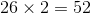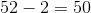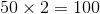Therefore (b) is greater.

### Example Question #182 : Data Analysis And Probability

A bag hasblue,green,red, andpurple balls.

Quantity A: The probability of choosingred ball andblue ball after two consecutive picks, with replacement.

Quantity B: The probability of choosingblue orred ball on one pick.

The relationship cannot be determined from the information given.

The two quantities are equal.

Quantity B is greater.

Quantity A is greater.

Quantity B is greater.

Explanation:

Quantity A: There are a total ofballs, so the probabilty of choosingred ball ANDblue ball is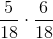, which gives us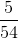.

Quantity B: The probability of choosingblue ball ORred ball is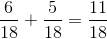.

Quantity B is larger.

### Example Question #22 : How To Find The Missing Part Of A List

If Joe flips a coin twice, what is the probability that at least one tail will occur?There are four possible outcomes (with H being heads and T being tails): HH, HT, TT, TH. There are three outcomes that contain a tail: TH, HT, and TT. Therefore the probaility that at least one flip will show tails is.